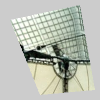# texture_mode()#

Sets the coordinate space for texture mapping.

## Examples#```def setup():
py5.size(100, 100, py5.P3D)
py5.no_stroke()
py5.texture_mode(py5.IMAGE)
py5.begin_shape()
py5.texture(img)
py5.vertex(10, 20, 0, 0)
py5.vertex(80, 5, 100, 0)
py5.vertex(95, 90, 100, 100)
py5.vertex(40, 95, 0, 100)
py5.end_shape()
``````def setup():
py5.size(100, 100, py5.P3D)
py5.no_stroke()
py5.texture_mode(py5.NORMAL)
py5.begin_shape()
py5.texture(img)
py5.vertex(10, 20, 0, 0)
py5.vertex(80, 5, 1, 0)
py5.vertex(95, 90, 1, 1)
py5.vertex(40, 95, 0, 1)
py5.end_shape()
```

## Description#

Sets the coordinate space for texture mapping. The default mode is `IMAGE`, which refers to the actual pixel coordinates of the image. `NORMAL` refers to a normalized space of values ranging from 0 to 1. This function only works with the `P2D` and `P3D` renderers.

With `IMAGE`, if an image is 100 x 200 pixels, mapping the image onto the entire size of a quad would require the points (0,0) (100,0) (100,200) (0,200). The same mapping in `NORMAL` is (0,0) (1,0) (1,1) (0,1).

Underlying Processing method: textureMode

## Signatures#

```texture_mode(
mode: int,  # either IMAGE or NORMAL
/,
) -> None
```

Updated on September 01, 2022 16:36:02pm UTC